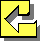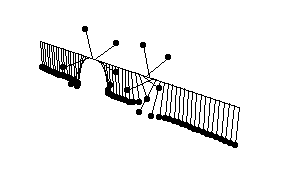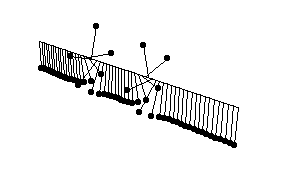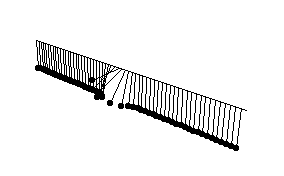# Many Faces of Solitons

### Kanehisa Takasaki (Kyoto University)

Entrance -- KdV equation -- Modified KdV equation -- Sine-Gordon equationEntrance of This Exhibition

## Sine-Gordon equation utt - uxx + sin u = 0

The term "sine-Gordon equation" is presumably a kind of joke, obviously originating in the name of the "Klein-Gordon equation" in relativistic field theories. As this name shows, this equation is a relativistic nonlinear equation in 1+1 dimensional space-time. Its precursor, just the KdV equation, can be found in the 19th century mathematics (Darboux's work on surface geometry). The sine-Gordon equation, too, has a wide range of applications in physics, not only in relativistic field theories but in solid-state physics, nonlinear optics, etc.

In order to visualize solutions, we use a coupled pendulum model. This is a mechanical model consisting of an elastic wire (or, rather, a straight spring) attached with perpendicular rods in an equal spacing. The rods behave as a pendulum receiving an angular force from the two neighboring rods through twist of the wire. (One can do a cheaper experiment using a rubber string and needles in place of a wire and rods.) In the limit as the spacing of rods tends to zero, this mechanical system approaches the sine-Gordon equation.

Soliton solutions of the sine-Gordon equation are far richer than those of the KdV and modified KdV equations. Even the 1-solution soliton solution consists of two different cases -- "kink" and "anti-kink". A kink is a solution whose boundary values at the left infinity is 0 and at the right infinity is 2pi; the boundary values of an anti-kink is 0 and -2pi, respectively. More intuitively, the chain of pendulums, in both cases, winds up once around the wire, but in an opposite direction. Similarly, 2-soliton solutions can be classified into several distinct cases -- collision of two "kinks", collision of two "anti-kinks", collision of a "kink" and "anti-kink", and a kind of "bound state" called "breather solution". The last one is rather hard to explain, but the animation will clearly show how it behaves.

• kink-antikink collision [gif]
Here is an animation of kink-anti-kink collision. The kink and the anti-kink are given the same speed and proceed in an opposite direction. Note that the twist of the pendulum chain disappears at a moment (t = 0) during collision.[kink-antikink solution before collision (coupled pendulum model)]
solution: u = 4*Arctan[ (p*Sinh[Sqrt[p^2 - 1]*t]) / (Sqrt[p^2 - 1]*Cosh[p*x]) ].
parameters: p = 2.

• kink-kink collision [gif]
Here is an animation of kink-kink collision. Since the kinks are twisted in the same direction, the pendulum chain remains twisted (twice) during collision. It is interesting that the two kinks look like "repelled" rather than collide. (I never imagined this phenomena until I made this animation!)[kink-kink solution before collision (coupled pendulum model)]
solution: u = 4*Arctan[ (Sqrt[p^2 -1]*Sinh[p*x]) / (p*Cosh[Sqrt[p^2 - 1]*t]) ].
parameters: p = 2.

• breather solution [gif]
Here is an animation of the "breather solution". This name originates in the behavior of its profile, which repeats regularly oscillating upwards and downwards, thereby looking like breathing. In the pendulum model, this behavior is nothing but a localized collective oscillation, as you see in the animation.[breather solution (coupled pendulum model)]
solution: u = 4*Arctan[ (p*Sin[Sqrt[p^2 +1]*t]) / (Sqrt[p^2 + 1]*Cosh[p*x]) ].
parameters: p = 2.